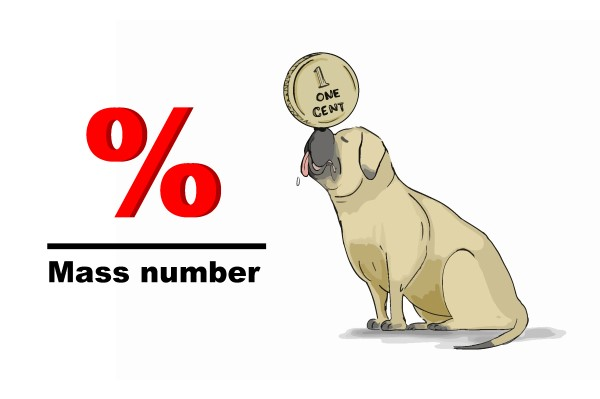# 3) Working out empirical formula

If you know the % components within a compound and want the formula, then divide all the percentages by the mass number of each element and you will get a series of numbers proportional to the number of atoms.The cent (percentage) is balanced on the nose of the bull mastiff (mass number).

### Example 1

What is the empirical formula of the compound that contains 11.2% hydrogen and 88.8% oxygen by mass?

Mass number of hydrogen = 1

Mass number of oxygen = 16

Remember divide % by mass number

Hydrogen       Oxygen

11.2%
1
:    88.8%
16

There is a ratio of:

Hydrogen: Oxygen

11.2: 5.55

Now all we have to do is work out a whole number ratio. To do this, divide the large number by the small number:

11.2
5.55
= 2

Therefore 11.2 (hydrogen): 5.55 (oxygen) is the same as 2:1.

The formula for the compound is H2O.

Below is an alternative way of tackling the problem. However, we believe our method is much easier.

What is the empirical formula of the compound that contains 11.2% hydrogen and 88.8% oxygen by mass?

1. Write what you know

%H = 11.2%                 1 mole of hydrogen = 1g

%O = 88.8%                 1 mole of oxygen = 16g

For every 100g of the compound there will be 11.2g of hydrogen and 88.8g of oxygen.

2. Calculate the number of moles of hydrogen and oxygen within 100g of the compound.

So the number of moles of each element within the compound is calculated as:

11.2
1
= 11.2 moles of hydrogen

88.8
16
= 5.6 moles of oxygen

3. Use molar ratio to work out empirical formula of the compound

The molar ratio of hydrogen to oxygen is therefore 11.2 : 5.6 = 2:1 so there are two hydrogen atoms to every oxygen atoms in the compound. Meaning the formula of the compound is H2O.

### Example 2

What is the empirical formula of the compound that contains 40% sulphur and 60% oxygen by mass?

Mass number of sulphur = 32

Mass number of oxygen = 16

Remember divide % by mass number

Sulphur           Oxygen

40%
32
: 60%
16

The ratio is      Sulphur: Oxygen

1.25: 3.75

Now all we have to do is work out a whole number ratio: divide the large number by the small number:

3.75
1.25
= 3

Therefore 1.25 (sulphur): 3.75 (oxygen) is the same as 1:3

So the empirical formula is SO3. Easy isn’t it!

Example 3

Elemental analysis showed 72.36% iron and 27.64% oxygen by mass. What is the empirical formula?

Mass number of iron = 56

Mass number of oxygen = 16

Remember divide % by mass number

Iron              Oxygen

72.36%
56
: 27.64
16

The ratio is:

Iron: Oxygen

1.292:  1.727

Now all we have to do is work out a whole number ratio: divide the large number by the small number:

1.727
1.292
= 1.33

Therefore,

Iron: Oxygen

1:         1.33

Try multiplying by 2 to get a whole number; if that doesn’t work try multiplying by 3 and if that doesn’t work, try multiplying by 4 etc.

In this case we can multiply by 3 to get

Iron: Oxygen

3: 4

So the empirical formula of the compound is Fe3O4.   Easy isn’t it!

### Example 4

Elemental analysis showed 41.09% K, 33.68% S and 25.22% O by mass, what is the formula?

Mass number of potassium = 39

Mass number of sulphur = 32

Mass number of oxygen = 16

Remember divide % by mass number

Potassium       Sulphur           Oxygen

41.09%
39
: 33.68%
32
: 25.22%
16

1.053 : 1.053 : 1.576

1 : 1 : 1.5

2 : 2 : 3

Therefore the formula is K2S2O3

### Example 5

A compound contains 92.26% carbon and 7.74% hydrogen by mass and has a molar mass of 78.0 g/mol. What is the molecular formula?

Mass number of carbon = 12

Mass number of hydrogen = 1

Remember divide % by mass number

Carbon : Hydrogen

92.26%
12
: 7.74%
1

7.7 : 7.7

1 : 1

C : H

But, the weight of 1 mole of CH is as follows:

C = there are 12 protons and neutrons in carbon, each weighing 1
6 x 1023
g

H = There are is 1 proton in hydrogen, weighing 1
6 x 1023
g

The total weight of CH is therefore (12+1) x 1
6 x 1023
g

The weight of 1 mole of CH is 6 x 1023 x 13 x 1
6 x 1023
g

The weight of 1 mole of CH is 13, but because the molar mass = 78.0 g/mole = 78
13
= 6, the formula must be (CH)6 or C6H6.